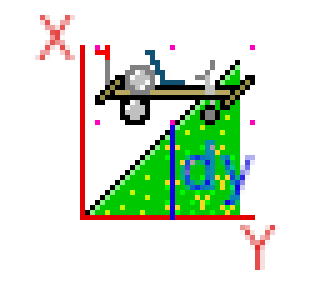# Slope Collision

## Recommended Posts

Posted from my site.

Slope Collision Detection

So one big thing that would make Trail Hogs a better game is to be able to go up and down hills because that is what I do when I ride the bike. So to accomplish this I will need to learn how to do slope collision detection in 2D at least for now!

Coordinates

The first thing to think about when dealing with slope collision are the coordinates. After thinking about this for a while it appears that only the Y coordinate needs to be modified when colliding with a slope. The X coordinate stays the same. The Y coordinate is calculated by multiplying a ratio to it. For example, a 45 degree slope will have a rise to run ratio of one. This means that both the X and Y coordinates are the same relative to the slope.As we can see in the image there is a central point in the player sprite (the bike) which is marked in pink. Our goal is to determine the point where this part of the sprite would make a collision with the slope. To calculate that coordinate you need to know the angle of the slope. That would be hard coded to the meta data of the slope sprite. This slope is 45 degrees. So to calculate that Y coordinate we need to use the distance that the sprite is inside of the slope.

```var dx
var dy
var ratio

set #[ratio] to 1
set #[dx] to #[sprite]:x - #[sprites]:[slope]:x
set #[dy] to #[dx] * ##[ratio]```

We can further augment this coordinate by adding the slope's Y coordinate to it.

```var slope_hit_y

set #[slope_hit_y] to #[sprites]:[slope]:y + #[sprites]:[slope]:height - #[dy]```

This will set the coordinate that the player needs to hit. Because of the top down Y coordinate system we need there is a need to subtract #dx# from the height.

If you have a shallower slope like 22 degrees you would use a different ratio to calculate the Y coordinate. A ratio for 22 degrees would be 1/2. To calculate the new Y coordinate you would do:

```set #[ratio] to 2
set #[dy] to #[dx] / #[ratio]```

This will give you a half coordinate of X which is correct for Y.

Similarly, for a 66 degree slope there would be ratio of 1 and 1/2. To calculate this you would do:

`set #[dy] to #[dx] / 2 + #[dx]`

C-Lesh does not follow order of operations so the calculation must be written this way!

The Sprite

For the sprite we need to look at what angle the slope is and the sprite should be rotated to follow that. This rotation should be kept when the sprite is jumping up. It should be set when the sprite hits the slope. Also pay attention to negative angles which are backwards slopes.

## Join the conversation

You can post now and register later. If you have an account, sign in now to post with your account.
Note: Your post will require moderator approval before it will be visible.×   Pasted as rich text.   Paste as plain text instead

Only 75 emoji are allowed.